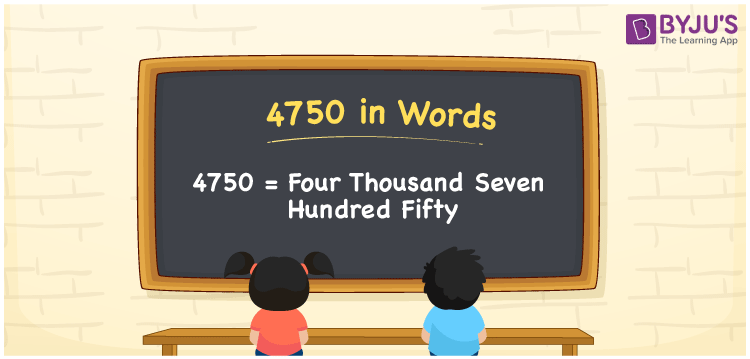# 4750 in Words

4750 in words is four thousand seven hundred fifty. In both, the system of numerals such as the Indian and the International place value system, the number 4750 is expressed as “Four Thousand Seven Hundred and Fifty”. Hence, the place value system is found to be useful while converting 4750 into words. Now, let us discuss the spelling of 4750 in English words with a detailed explanation.

 4750 in Words: Four Thousand Seven Hundred Fifty. Four Thousand Seven Hundred Fifty in Numerical Form: 4750.

## 4750 in English Words## How to Write 4750 in Words?

The number 4750 is expressed in the place value chart as follows:

 Thousands Hundreds Tens Ones 4 7 5 0

The expanded form of 4750 is as follows:

= 4 × Thousand + 7 × Hundred + 5 × Ten + 0 × One

= 4 × 1000 + 7 × 100 + 5 × 10

= 4000 + 700 + 50

= 4750

= four thousand seven hundred fifty

Hence, 4750 in words is four thousand seven hundred fifty.

4750 in words – Four thousand seven hundred fifty

Is 4750 an odd number? – No

Is 4750 an even number? – Yes

Is 4750 a perfect square number? – No

Is 4750 a perfect cube number? – No

Is 4750 a prime number? – No

Is 4750 a composite number? – Yes

## Frequently Asked Questions on 4750 in Words

Q1

### How to write 4750 in words?

4750 in words is four thousand seven hundred fifty.

Q2

### Simplify 4000 + 750, and express it in words.

Simplifying 4000 + 750, we get 4750. Hence, 4750 in words is four thousand seven hundred fifty.

Q3

### Is 4750 a composite number?

Yes, 4750 is a composite number.